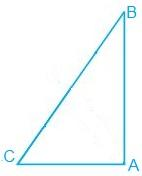# ABC is a right-angled triangle in which A = 90° and AB = AC. Find B and C.#### Solution

Given

ABC is a right-angled triangle in which ∠A = 90° and AB = AC.

Find out

∠B=? and ∠C?

In ∆ABC,

AB=AC

∴∠B=∠C

∠A+∠B+∠C=180°

90°+∠B+∠B=180°

2∠B=180°-90°

∠B=(90°)/2=45°

Therefore, ∠B=45° and ∠C =45°